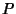## Pedal Circle

The pedal Circle of a pointin a Triangle is the Circle through the feet of the perpendiculars fromto the sides of the Triangle (the Circumcircle about the Pedal Triangle). Whenis on a side of the Triangle, the line between the two perpendiculars is called the Pedal Line. Given four points, no three of which are Collinear, then the four Pedal Circles of each point for the Triangle formed by the other three have a common point through which the Nine-Point Circles of the four Triangles pass. The radius of the pedal circle of a pointis(Johnson 1929, p. 141).